What Is Connectionism?

István S. N. Berkeley Ph.D.

Bibliography

Connectionism is a style of modeling based upon networks of interconnected simple processing devices. This style of modeling goes by a number of other names too. Connectionist models are also sometimes referred to as 'Parallel Distributed Processing' (or PDP for short) models or networks.1 Connectionist systems are also sometimes referred to as 'neural networks' (abbreviated to NNs) or 'artificial neural networks' (abbreviated to ANNs). Although there may be some rhetorical appeal to this neural nomenclature, it is in fact misleading as connectionist networks are commonly significantly dissimilar to neurological systems. For this reason, I will avoid using this terminology, other than in direct quotations. Instead, I will follow the practice I have adopted above and use 'connectionist' as my primary term for systems of this kind.

The basic components of a connectionist system are as follows;

1. A set of processing units
2. A set of modifiable connections between units
3. A learning procedure (optional)

I will describe each of these components in turn. Readers who require further technical details should consult the general framework for connectionist systems described by Rumelhart, Hinton and McClelland (1987).

Processing Units

Processing units are the basic building blocks from which connectionist systems are constructed. These units are responsible for performing the processing which goes on within a connectionist network. The precise details of the processing which goes on within a particular unit depends upon the functional subcomponents of the unit. There are three crucial subcomponents. These are,

• a) The net2 input function
• b) The activation function
• c) The output function

The various components of a processing unit can be represented as follows,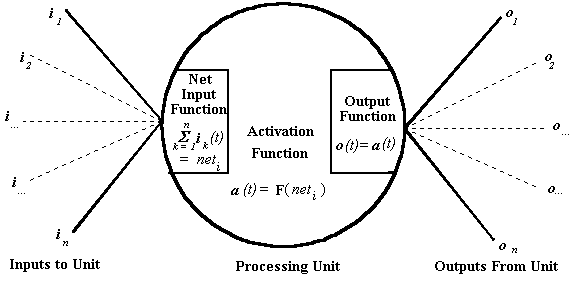Figure 3-1

The net input function of a processing unit determines the total signal that a particular unit receives. The net input function takes as input the signal which a unit receives from all sources (ii-m), including the other units which it is connected to. It is often the case that the net input function of a unit is relatively simple. Commonly, the net input function for a unit will just sum the of the input signals the unit receives at a particular time (t).

The activation function of a particular unit determines the internal activity of the unit, depending upon the net input (as determined by the net input function) that the unit receives. There are many different kinds of activation functions which particular units can employ. The 'type' of a particular unit is determined by its activation function. Perhaps the simplest kind of activation function is illustrated below,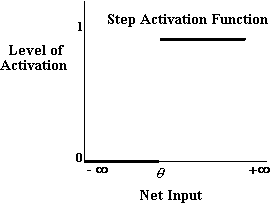Figure 3-2

Activation functions such as this act rather like switches and are sometimes called 'step functions'. If the net input to a unit employing such an activation function is greater than some threshold value, q, the unit becomes fully active.3 If the net input is below this level, the processing unit is totally inactive. The activation function, aj, for such a unit, j, can be expressed more formally as follows;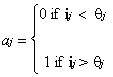where ij is the net input received by the unit at time t and qj is the threshold value for unit j.

Activation functions of this kind were used in the very earliest days of network research. Unfortunately though they are subject to certain significant limitations (see Minsky & Papert 1968). In particular, it is not possible to train networks which employ this kind of unit arranged into more than two layers.

Currently, within the domain of trainable networks, by far the most common kind of processing unit employed by connectionists is what Ballard (1986) has called an 'integration device'. The logistic function described by Rumelhart et al (1986a: pp. 324-325), for example, is an instance of an integration device. Integration devices have a sigmoidal activation function, similar to the one illustrated below, and can be described as a continuous approximation of a step function.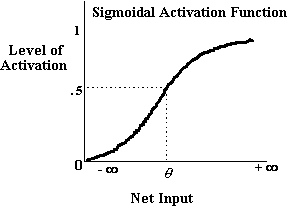Figure 3-3

The activation function, aj, for a unit, j, of this variety, receiving net input ij is;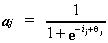Integration devices include in their activation function something known as 'bias'. Bias serves to alter the level of input to a unit which is needed for that unit to become active and is therefore analogous to the threshold of a step function. In more technical terms, bias serves to translate the activation function along an axis representing net input, thereby altering the location of the activation function in net input space. The j term in the logistic equation is the bias term of that activation function.

One important feature of sigmoidal activation functions is that they be differentiable. The reason this is important is that it make it possible to train networks with more than two layers of processing units, using powerful learning rules such as the generalized delta rule, described by Rumelhart, Hinton and Williams (1986a: pp. 322-328). This ability to train networks with multiple layers has greatly increased the power of networks.

Although integration device units are arguably the most commonly employed unit type in trainable networks at the current time, other activation functions have also been explored. Recently, Dawson and Schopflocher (1992) have described a kind of processing unit which they call, following Ballard's (1986) terminology, a 'value unit' . Value units employ a Gaussian activation function, such as the one below,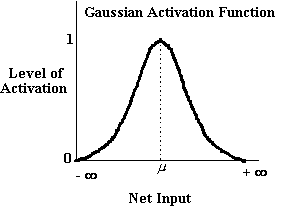Figure 3-4

The activation function, aj, for a unit, j, of this variety, receiving net input ij is;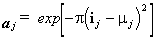As the net input, ij, to a value unit increases, the level of activation of the unit, aj, increases, but only up to a certain point, j. When ij = j, the activation aj is maximized and has a value of 1. If the unit receives net input greater than j, the activation of the unit begins to decline again, down to 0. As a consequence of having this kind of activation function, value units will only generate strong activation for a narrow range of net inputs. Value units, like integration devices, can be used to construct trainable multilayered networks.

A unit in a connectionist network typically sends a signal to other units in the network or to outside the network. The signal that a unit sends out is determined by the output function. The output function depends upon the state of activation of the unit. It is common practice, at the current time, that the output function of a particular unit is such that it just sends out a signal equivalent to its activation value. However, there is no theoretical reason why this must necessarily be the case.

Modifiable Connections

In order for a particular connectionist network to process information, the units within the network need to be connected together. It is via these connections that the units communicate with one another. The connections within a network are usually 'weighted'. The weight of a connection determines the amount of the signal input into the connection which will be passed between units. Connection weights (sometimes also called 'connection strengths') are positive or negative real numerical values. The amount of input a particular connection supplies to a unit to which it is connected is the value of the result of the output function of the sending unit, multiplied by the weight of the connection.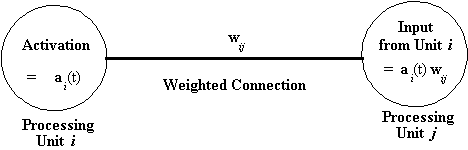Figure 3-5

In principle, there is no limit to the number or pattern of connections which a particular unit may have. Units can have weighted connections with themselves and there can even be loops or cycles of connections. However, for current purposes there is no need to explore such complexities. Instead, attention will be limited to simple three layered systems like the one illustrated below.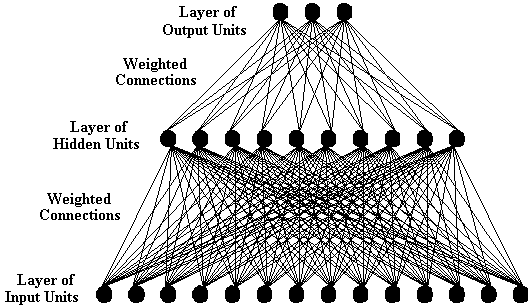Figure 3-6

If particular processing units within a system can receive inputs from sources external to the network itself, then these units are usually called input units. Alternatively, if particular processing units can send signals outside the network itself, then these units are usually called output units. Finally, processing units which can only directly communicate with other units within the network (i.e. units which have no direct inputs or outputs which are external to the network) are usually called hidden units. Layers of hidden units are not an essential feature of networks, although many networks require a single layer of hidden units to solve a particular problems. It is also the case that there is no reason why a network should just have a single layer of hidden units. For example, a network described by Bechtel and Abrahamsen (1991: p. 169) has two layers of hidden units.

Learning Rules

A learning rule is an algorithm which can be used to make changes to strengths of the weights of the connections between processing units. Whereas all connectionist systems have processing units and patterns of connections between the units, not all systems have learning rules. Some networks (e.g. the Jets and Sharks Interactive Activation and Competition network, described in McClelland and Rumelhart (1988)) are built by hand (or 'hand-coded'). Hand-coded networks have the weights of the connections between the processing units set manually by the network's builder. However, in most connectionist networks a learning rule of some kind is employed. In this dissertation I will be concerned primarily with networks that employ learning rules.

A learning rule is used to modify the connection weights of a network so as (hopefully) to make the network better able to produce the appropriate response for a given set of inputs. Networks which use learning rules have to undergo training, in order for the learning rule to have an opportunity to set the connection weights. Training usually consists of the network being presented with patterns which represent the input stimuli at their input layer. It is common for connection weights to be set randomly prior to training.

For example, consider one of the most popular learning rules for connectionist networks, Rumelhart, Hinton and McClelland's (1986) generalized delta rule. When using this rule, the network is shown example patterns from a training set. The purpose of the generalized delta rule is to modify the network's connection weights in such a way that the network generates a desired response to each pattern in the training set.

More specifically, with the generalized delta rule learning proceeds by presenting one of the patterns from the training set to the network's input layer. This causes a signal to be sent to the hidden layer(s), which in turn results in a signal being sent to the output layer. In the generalized delta rule, the actual activation values of each output unit are compared to the activation values that are desired for the input pattern. The error for each output unit is the difference between its actual and desired activation. The generalized delta rule uses this error term to modify the weights of the connections that are directly attached to the output units. Error is then sent through these modified weights as a signal to the hidden units, which use this signal to compute their own error. The error computed at this stage is then used to modify the connection weights between the input units and the hidden units. In every case, when a weight is changed, the generalized delta rule guarantees that this change will reduce the network's error to the current input pattern.

Usually, the learning rule only makes small changes to the connections weights between the layers each time it is applied. As a result training often requires numerous presentations of the set of input patterns. By the repeated presentation of the training set and application of the learning rule, networks can learn to produce the correct responses to the set of inputs which make up the training set. Learning rules thus offer a means of producing networks with input/output mappings appropriate to particular tasks or problems. Each presentation of the set of input patterns and output patterns is known as an 'epoch' or a 'sweep'. When the network produces an output for each input pattern which is close enough (as determined by the experimenter) to the desired output for each pattern, training stops and the network is said to have 'converged'.

Bibliography

Notes

• 1) Although in current usage, the terms 'connectionist' and 'PDP' have effectively become synonyms, the two terms once had different meanings. Originally, so-called 'Connectionist' models were generally associated with Ballard's work at the University of Rochester. So-called 'PDP' models, on the other hand, were associated with the PDP Research Group of San Diego (for more details on the etymology of these terms, see Smolensky 1991: p. 225, fn. 5). I will follow what is now current practice and use the two terms as synonyms.

• 2) The term 'net' here is not meant as an abbreviation of the term 'network'. The intended sense is that of 'net' as opposed to 'gross'.

• 3) Note, the activation levels need not be 0 and 1. These values are employed merely for illustrative purposes.